# IB DP Physics: SL复习笔记2.4.3 Conservation of Linear Momentum

### Conservation of Linear Momentum

• The principle of conservation of linear momentum states:

The total momentum before a collision = the total momentum after a collision provided no external force acts

• Linear momentum is the momentum of an object that only moves in one dimension
• Momentum is a vector quantity
• This means oppositely-directed vectors can cancel each other out resulting in a net momentum of zero
• If after a collision an object starts to move in the opposite direction to which it was initially travelling, its velocity will now be negative
• Momentum, just like energy, is always conserved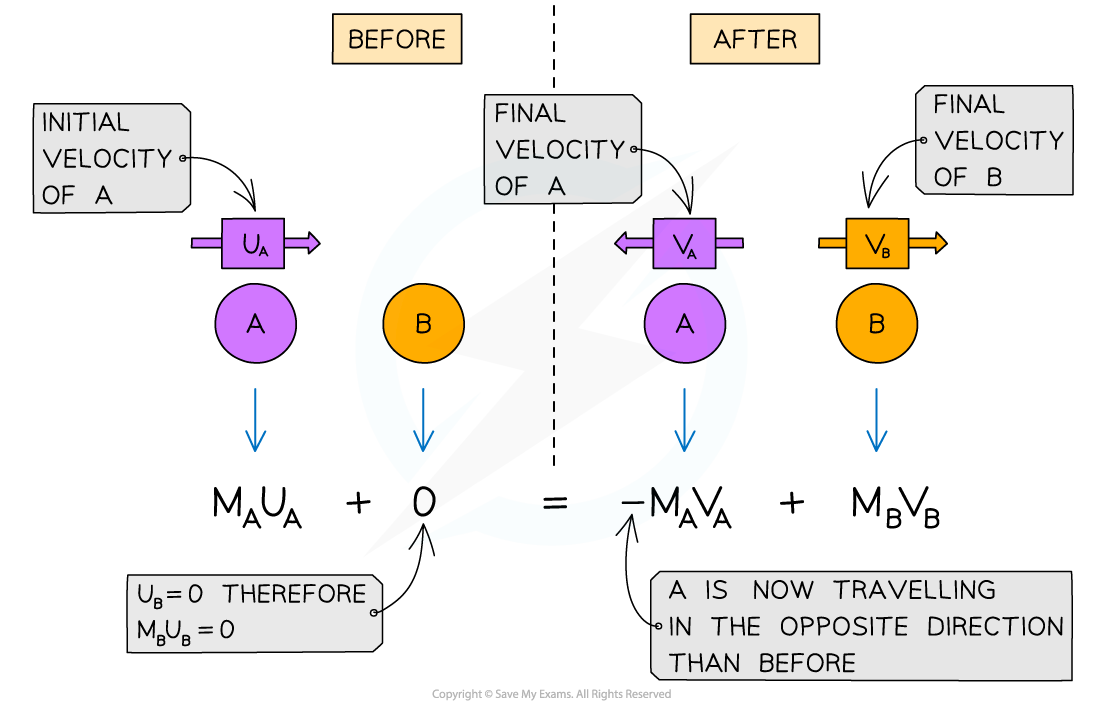The conservation of momentum for two objects A and B colliding then moving apart

#### External & Internal Forces

• External forces are forces that act on a system from outside of it e.g. friction and weight
• Internal forces are forces exchanged by the particles in the system e.g. tension in a string
• Forces that are internal or external will depend on the system itself
• For example, in a mass-spring system:
• The internal force is the spring force
• The external force is the weight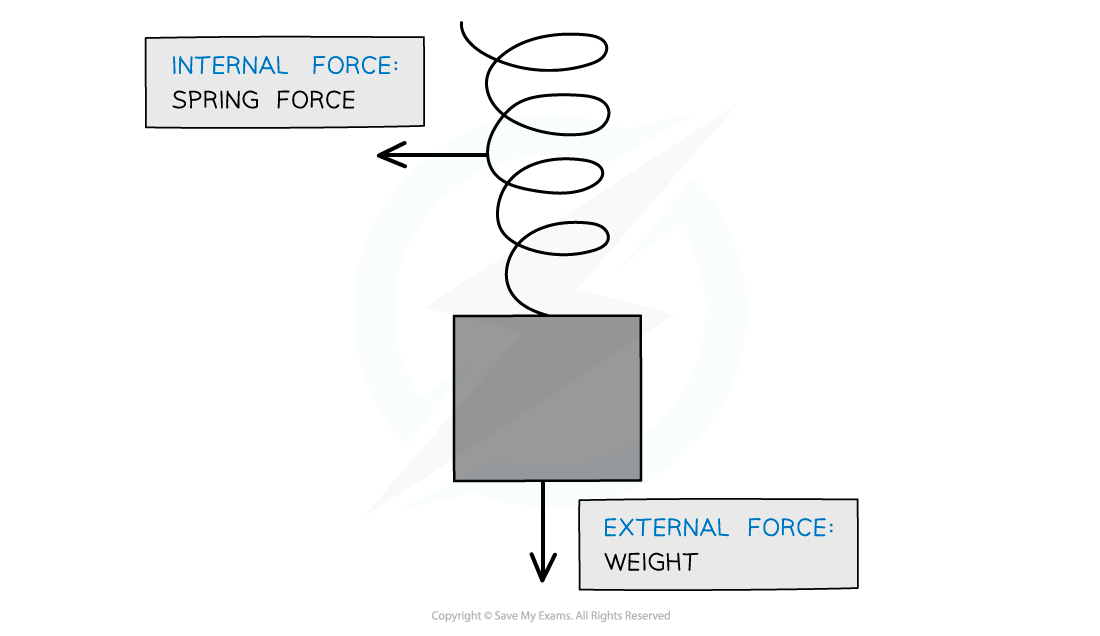Internal and external forces on a mass on a spring

• Systems with no external forces may be described as ‘closed’ or ‘isolated’
• These are keywords that refer to a system that is not affected by external forces
• For example, a swimmer diving from a boat:
• The diver will move forwards, and, to conserve momentum, the boat will move backwards
• This is because the momentum beforehand was zero and no external forces were present to affect the motion of the diver or the boat

#### Worked Example

Trolley A of mass 0.80 kg collides head-on with stationary trolley B whilst travelling at3.0 m s–1. Trolley B has twice the mass of trolley A. On impact, the trolleys stick together.

Using the conversation of momentum, calculate the common velocity of both trolleys after the collision.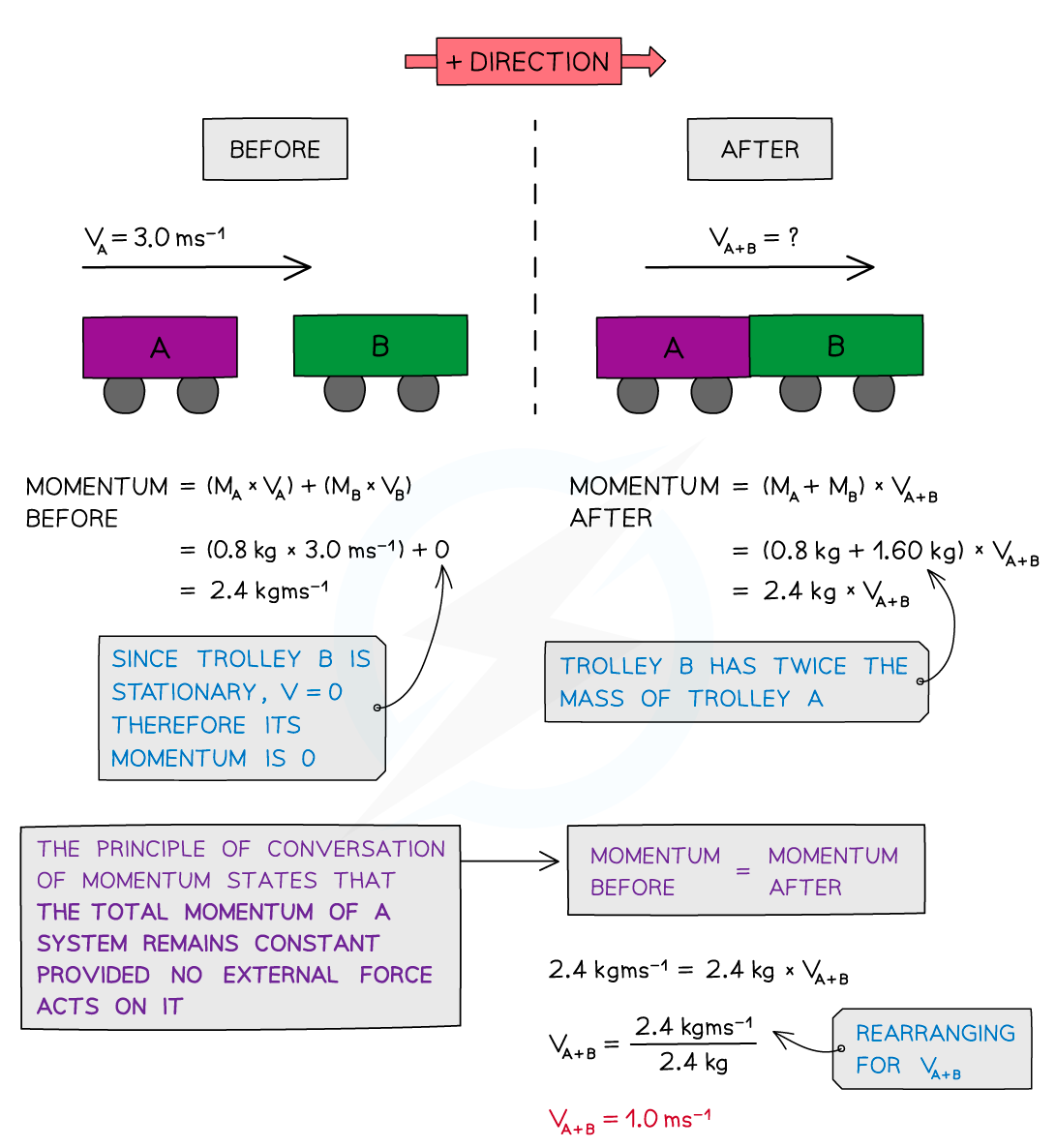#### Worked Example

The diagram shows a car and a van, just before and just after the car collided with the van, which is initially at rest.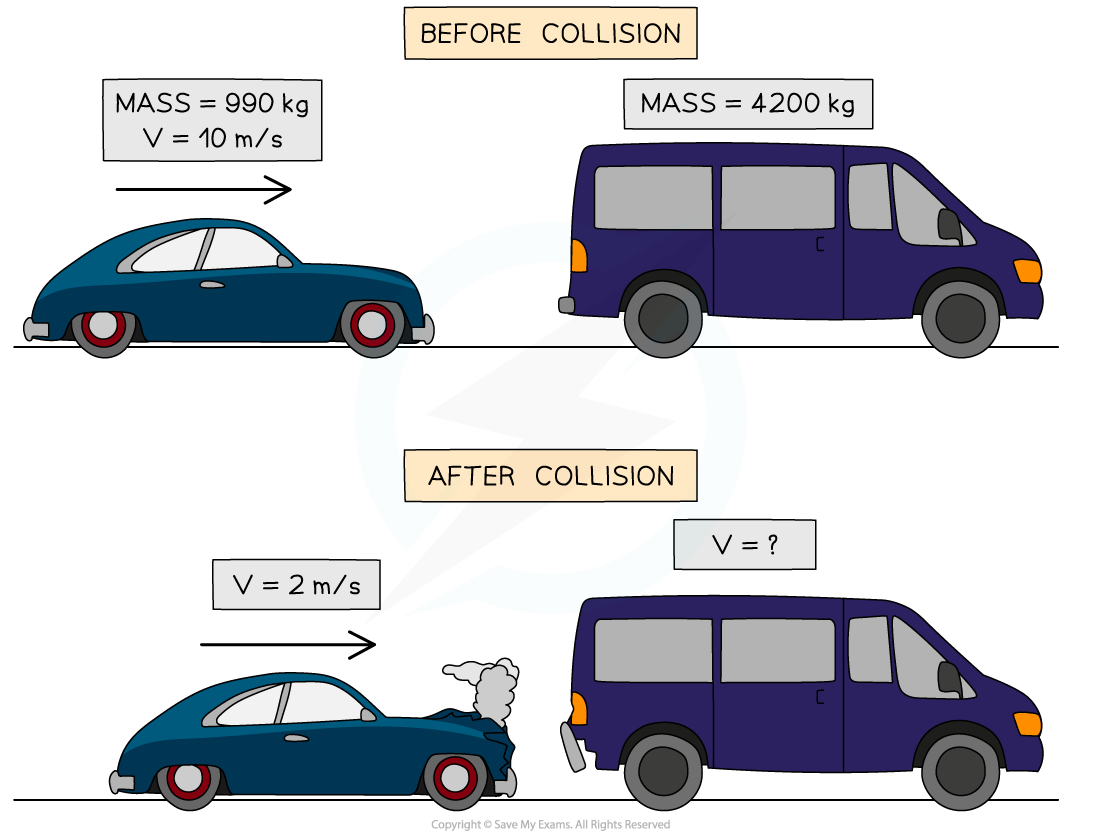Use the idea of conservation of momentum to calculate the velocity of the van when it is pushed forward by the collision.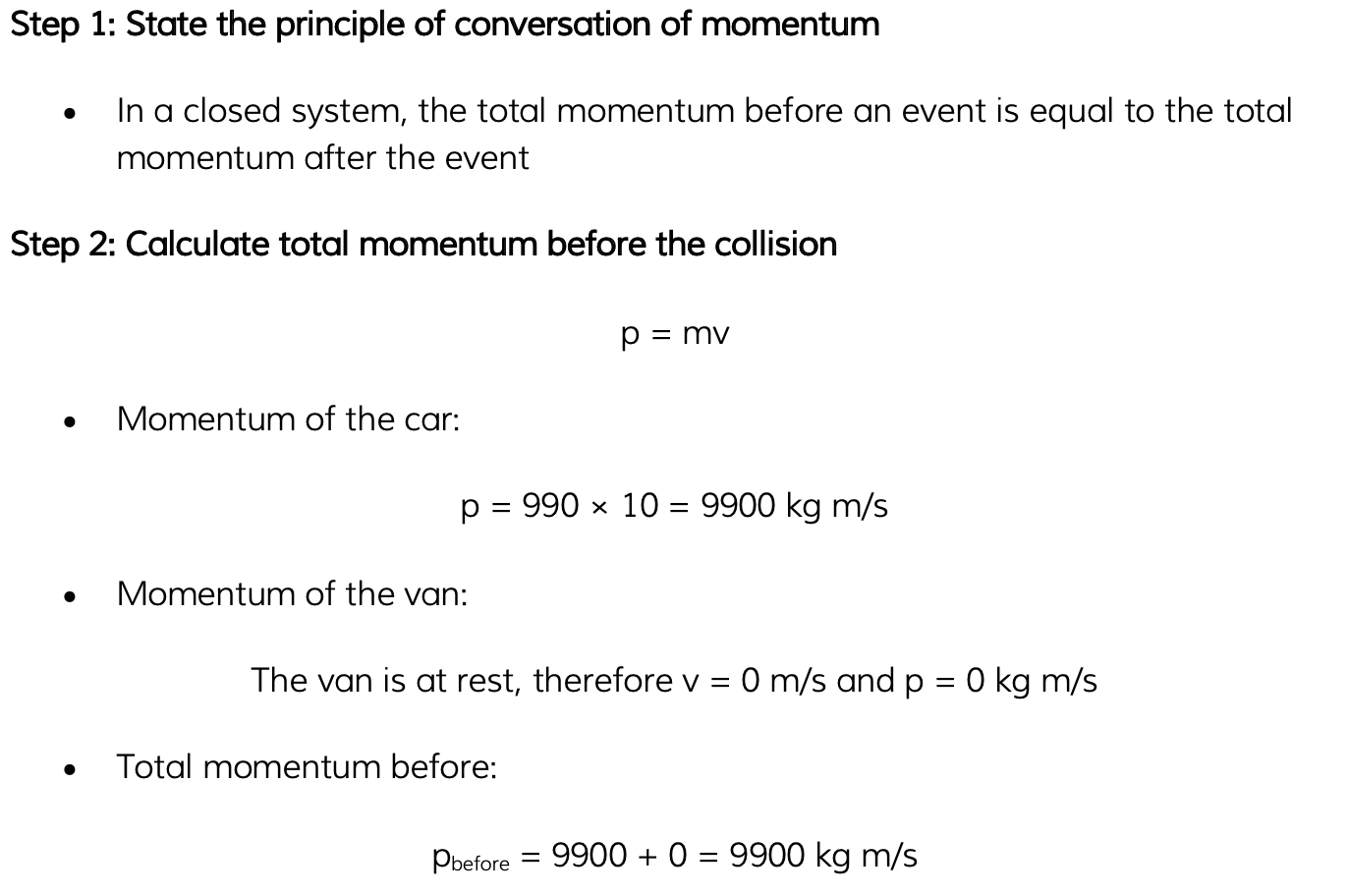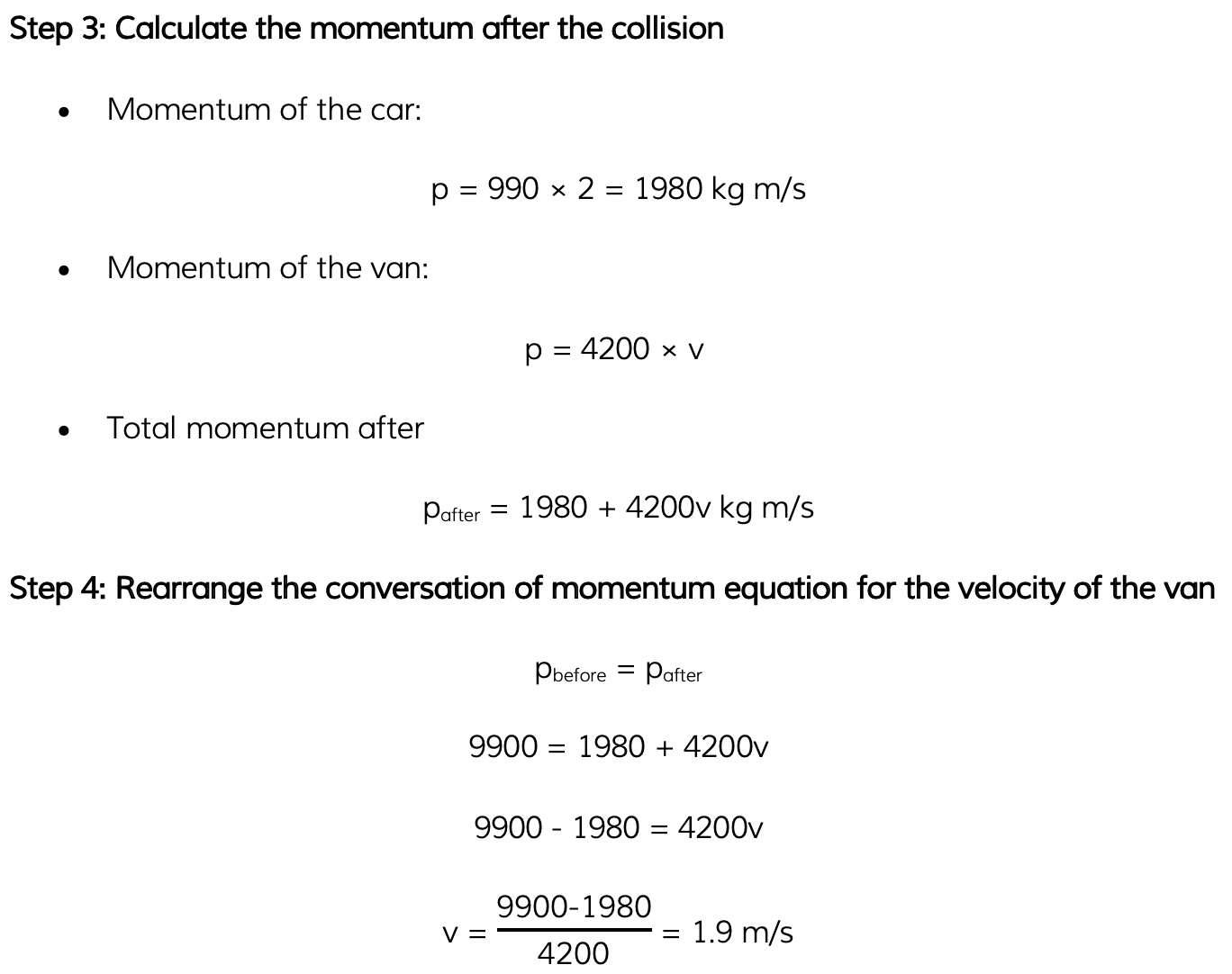#### Exam Tip

If it is not given in the question already, drawing a diagram of before and after helps keep track of all the masses and velocities (and directions) in the conversation of momentum questions.